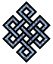fail2ban bad ip database: ip 35.227.34.247

| ip database | live view | stats | report | help | api key:

 ip: 35.227.34.247 hostname: 247.34.227.35.bc.googleusercontent.com country:[US] United States first reported: 20.11.2018 21:12.55 GMT+0200 last reported: 28.02.2019 08:44.58 GMT+0200 time period: 99d 11h 32m 03s total reports: 12 reported by: 3 host(s) filter(s): ssh (11) ssh (1) tor exit node no badips.com db Lookupport scan of '35.227.34.247':

[-hide]
```# Nmap 6.40 scan initiated Tue Nov 20 21:13:02 2018 as: /usr/bin/nmap -sU -sS -O 35.227.34.247
Nmap scan report for 247.34.227.35.bc.googleusercontent.com (35.227.34.247)
Host is up (0.092s latency).
Not shown: 1000 open|filtered ports, 996 filtered ports
PORT     STATE  SERVICE
22/tcp   open   ssh
80/tcp   closed http
443/tcp  closed https
3389/tcp closed ms-wbt-server
No exact OS matches for host (If you know what OS is running on it, see http://nmap.org/submit/ ).
TCP/IP fingerprint:
OS:SCAN(V=6.40%E=4%D=11/20%OT=22%CT=80%CU=%PV=N%G=Y%TM=5BF46B0E%P=x86_64-pc
OS:-linux-gnu)SEQ(SP=104%GCD=1%ISR=105%TI=Z%TS=A)SEQ(SP=104%GCD=1%ISR=105%T
OS:I=Z%CI=RI%TS=A)OPS(O1=M58CST11NW7%O2=M58CST11NW7%O3=M58CNNT11NW7%O4=M58C
OS:ST11NW7%O5=M58CST11NW7%O6=M58CST11)WIN(W1=6E00%W2=6E00%W3=6E00%W4=6E00%W
OS:5=6E00%W6=6E00)ECN(R=Y%DF=Y%TG=40%W=6EF0%O=M58CNNSNW7%CC=Y%Q=)T1(R=Y%DF=
OS:Y%TG=40%S=O%A=S+%F=AS%RD=0%Q=)T2(R=N)T3(R=N)T4(R=Y%DF=Y%TG=40%W=0%S=A%A=
OS:Z%F=R%O=%RD=0%Q=)T5(R=Y%DF=Y%TG=40%W=0%S=Z%A=S+%F=AR%O=%RD=0%Q=)T6(R=Y%D
OS:F=Y%TG=40%W=0%S=A%A=Z%F=R%O=%RD=0%Q=)T7(R=N)U1(R=N)IE(R=Y%DFI=N%TG=40%CD
OS:=S)

OS detection performed. Please report any incorrect results at http://nmap.org/submit/ .
# Nmap done at Tue Nov 20 21:14:06 2018 -- 1 IP address (1 host up) scanned in 65.67 seconds
```
```Σ = 76 | Δt = 0.0042879581451416s
```